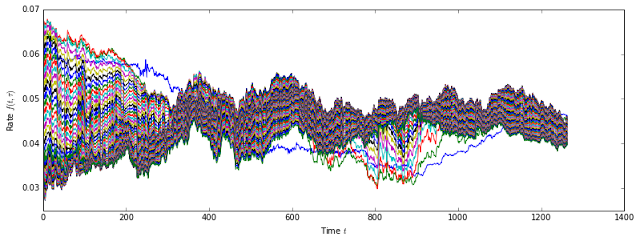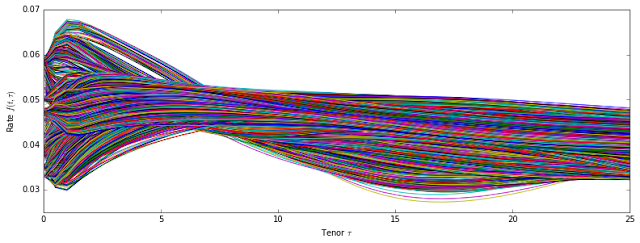## Sunday, November 13

### Heath Jarrow Morton Multi Factor Model

The IPython notebook which is subject of this post contains working implementation of a multi factor Heath Jarrow Morton (HJM) model. As most of the details are already described in the notebook itself, this article provides just brief summary.Yield Curve Data

The model is calibrated from historical daily yield curve data over the last five years. The yield curve structure contains 51 tenors ranging from 1M to 25Y.Evolution of daily historical yield curve data with 51 tenors over 5 years. Each line represents a different tenorEvolution of daily historical yield curve data with 51 tenors over 5 years. Each line represents different day in the past, while the horizontal axis represents tenor space.

Principal Component Analysis

The principal component analysis is then used to identify common factors which are driving movements in these rates. Typically 3-5 factors are sufficient to explain dynamics of the yield curve.

Volatility Fitting

Once the volatility factors are identified in historical data by PCA technique, cubic spline interpolator is used to fit these factors. These interpolators are then later used to generate discrete mesh of tenors for the purpose of Monte Carlo simulation.

Risk-neutral Drift Calculation

Drift function is calculated using numerical integration over fitted volatility functions

Monte Carlo Simulation

Monte Carlo Simulation with risk-neutral drift is used to generate evolution of yield curve for different simulation paths in order to price IR Caplet with strike 3%, expiring in one and maturing in two years.